# Retaining Walls 1 Function of retaining wall Retaining

• Slides: 43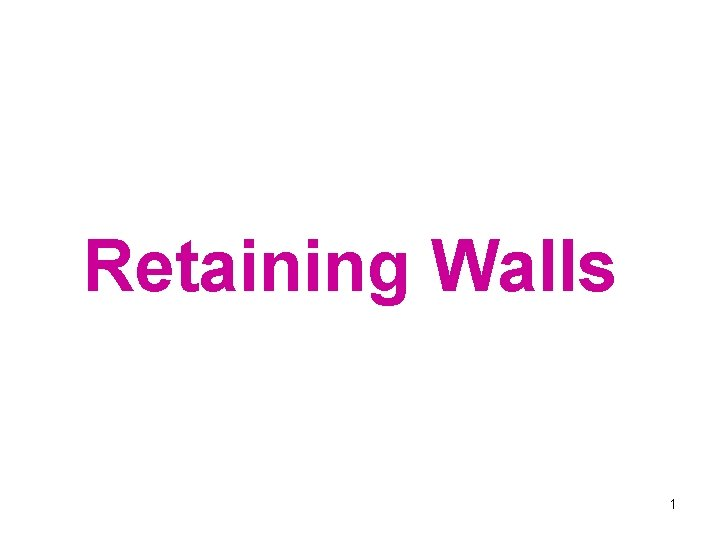Retaining Walls 1Function of retaining wall Retaining walls are used to hold back masses of earth or other loose material where conditions make it impossible to let those masses assume their natural slopes. Ø Such conditions occur when the width of an excavation, cut, or embankment is restricted by conditions of ownership, use of the structure, or economy. For example, in railway or highway construction the width of the right of way is fixed, and the cut or embankment must be contained within that width. ØSimilarly, the basement walls of the buildings must be located within the property and must retain the soil surrounding the base. 2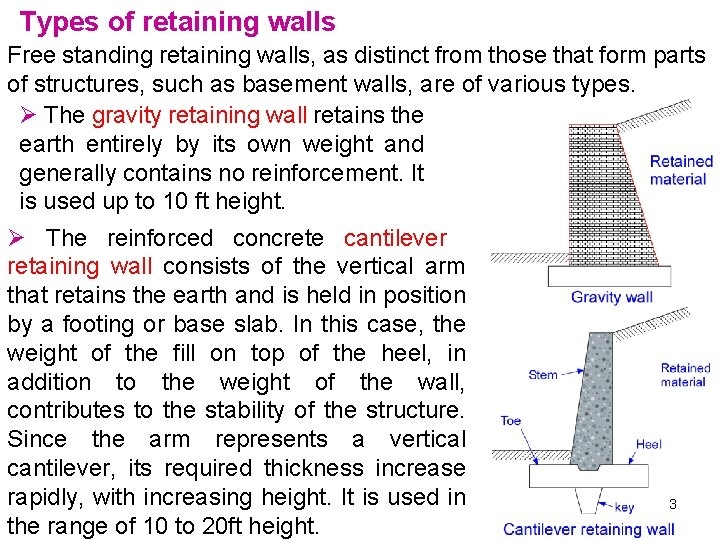Types of retaining walls Free standing retaining walls, as distinct from those that form parts of structures, such as basement walls, are of various types. Ø The gravity retaining wall retains the earth entirely by its own weight and generally contains no reinforcement. It is used up to 10 ft height. Ø The reinforced concrete cantilever retaining wall consists of the vertical arm that retains the earth and is held in position by a footing or base slab. In this case, the weight of the fill on top of the heel, in addition to the weight of the wall, contributes to the stability of the structure. Since the arm represents a vertical cantilever, its required thickness increase rapidly, with increasing height. It is used in the range of 10 to 20 ft height. 3Types of retaining walls In the counterfort wall the stem and base slab are tied together by counterforts which are transverse walls spaced at intervals and act as tension ties to support the stem wall. Counterforts are of half or larger heights. Counterfort walls are economical for heights over 25 ft. Property rights or other restrictions sometimes make it necessary to place the wall at the forward edge of the base slab, i. e. to omit the toe. Whenever it is possible, toe extensions of one-third to one -fourth of the width of the base provide a more economical 4 solution.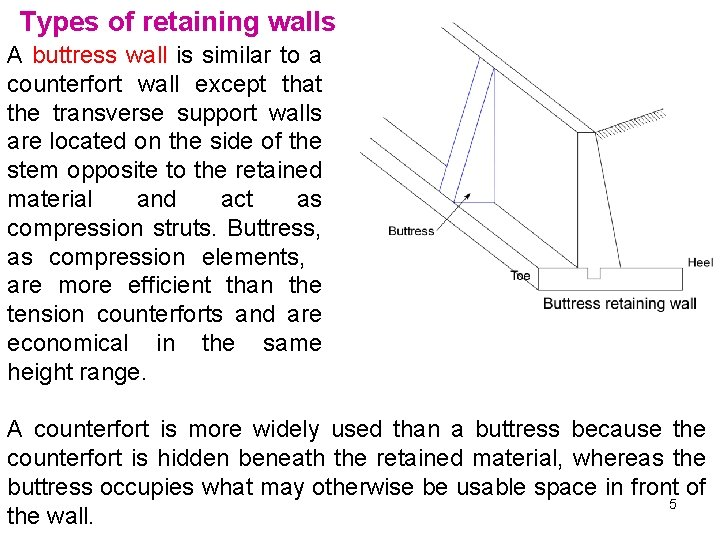Types of retaining walls A buttress wall is similar to a counterfort wall except that the transverse support walls are located on the side of the stem opposite to the retained material and act as compression struts. Buttress, as compression elements, are more efficient than the tension counterforts and are economical in the same height range. A counterfort is more widely used than a buttress because the counterfort is hidden beneath the retained material, whereas the buttress occupies what may otherwise be usable space in front of 5 the wall.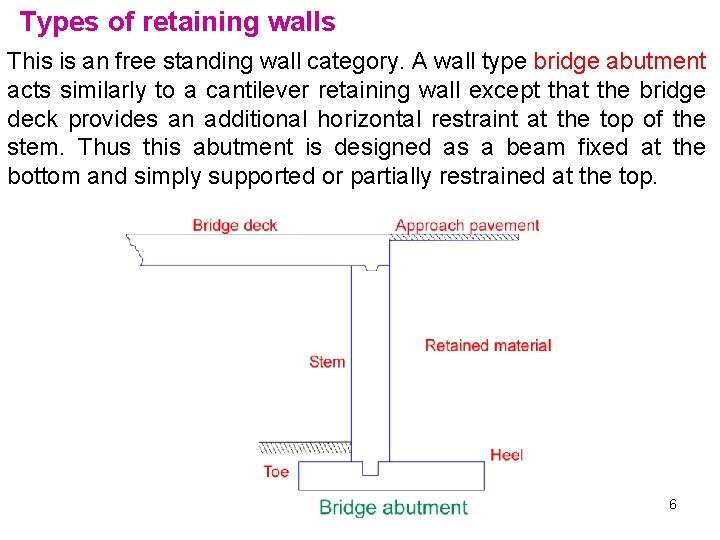Types of retaining walls This is an free standing wall category. A wall type bridge abutment acts similarly to a cantilever retaining wall except that the bridge deck provides an additional horizontal restraint at the top of the stem. Thus this abutment is designed as a beam fixed at the bottom and simply supported or partially restrained at the top. 6Earth Pressure Ø For liquid P=wwh, ww is the unit weight of water. ØSoil retaining structure Ph=Cowh Ø w is unit weight of the soil Ø C 0 is a constant known as the coefficient of earth pressure at rest According to Rankine, the coefficient for active and passive earth pressure are Ø For the case of horizontal surface =0 7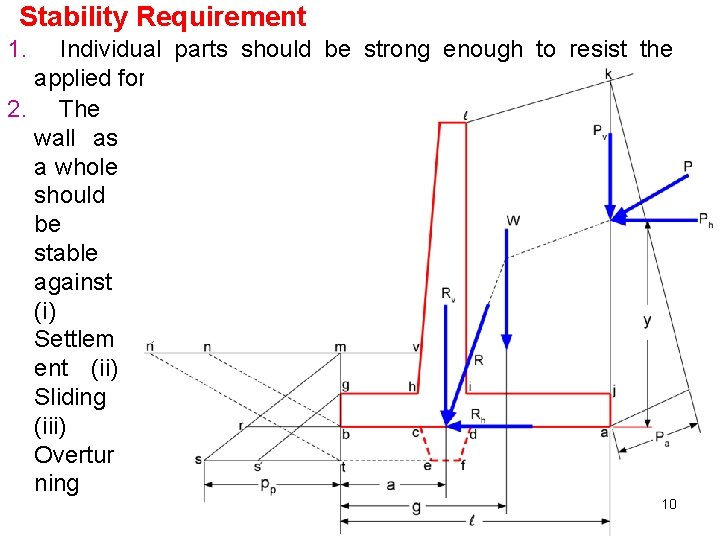Stability Requirement 1. Individual parts should be strong enough to resist the applied forces 2. The wall as a whole should be stable against (i) Settlem ent (ii) Sliding (iii) Overtur ning 10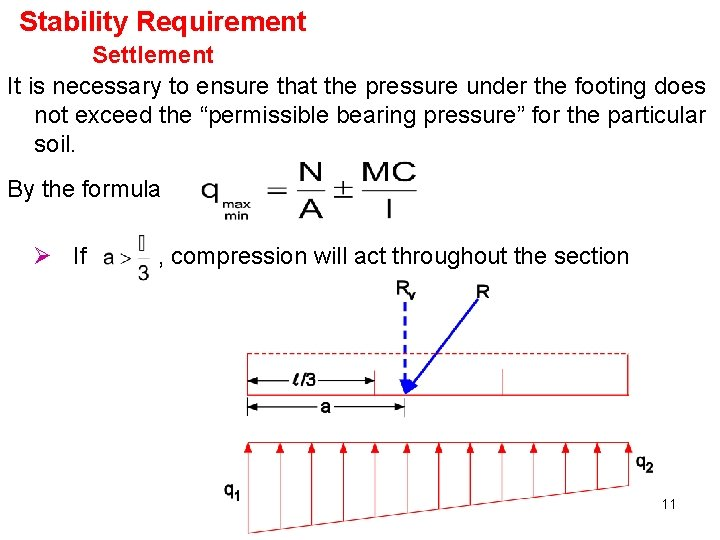Stability Requirement Settlement It is necessary to ensure that the pressure under the footing does not exceed the “permissible bearing pressure” for the particular soil. By the formula Ø If , compression will act throughout the section 11Stability Requirement Settlement 12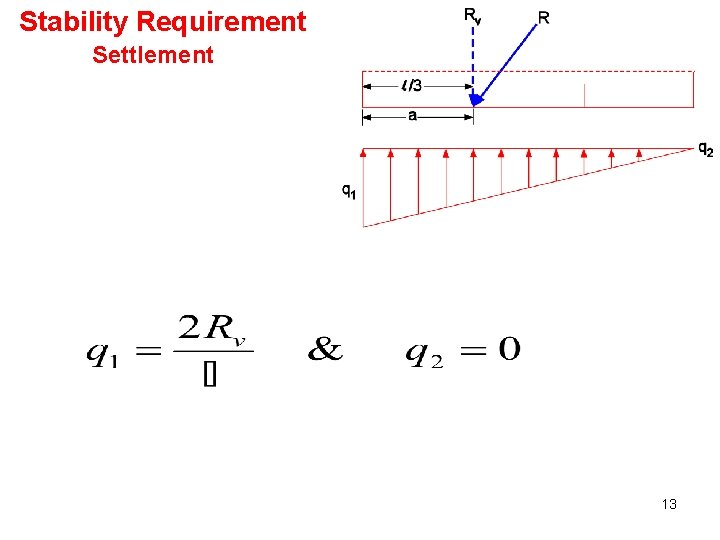Stability Requirement Settlement 13Stability Requirement Settlement 14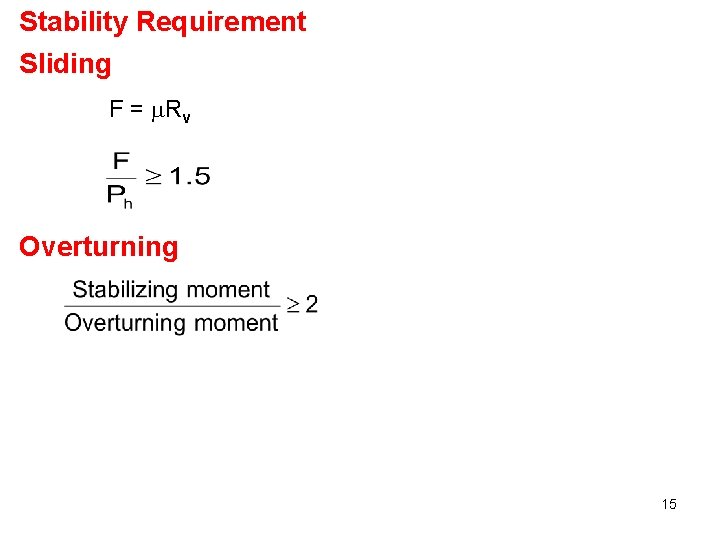Stability Requirement Sliding F = Rv Overturning 15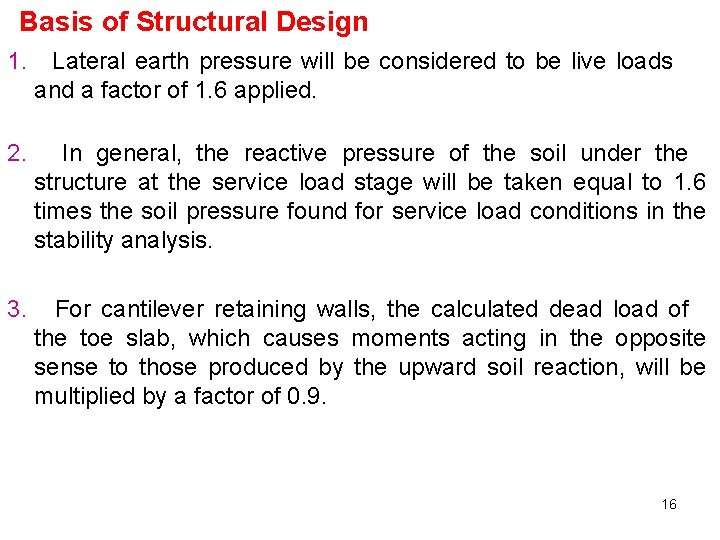Basis of Structural Design 1. Lateral earth pressure will be considered to be live loads and a factor of 1. 6 applied. 2. In general, the reactive pressure of the soil under the structure at the service load stage will be taken equal to 1. 6 times the soil pressure found for service load conditions in the stability analysis. 3. For cantilever retaining walls, the calculated dead load of the toe slab, which causes moments acting in the opposite sense to those produced by the upward soil reaction, will be multiplied by a factor of 0. 9. 16Basis of Structural Design 4. For the heel slab, the required moment capacity will be based on the dead load of the heel slab itself, plus the earth directly above it, both multiplied by 1. 2. 5. Surcharge, if resent, will be treated as live load with load factor of 1. 6. The upward pressure of the soil under the heel slab will be taken equal to zero, recognizing that for severe over load stage a non linear pressure distribution will probably be obtained, with most of the reaction concentrated near the toe. 17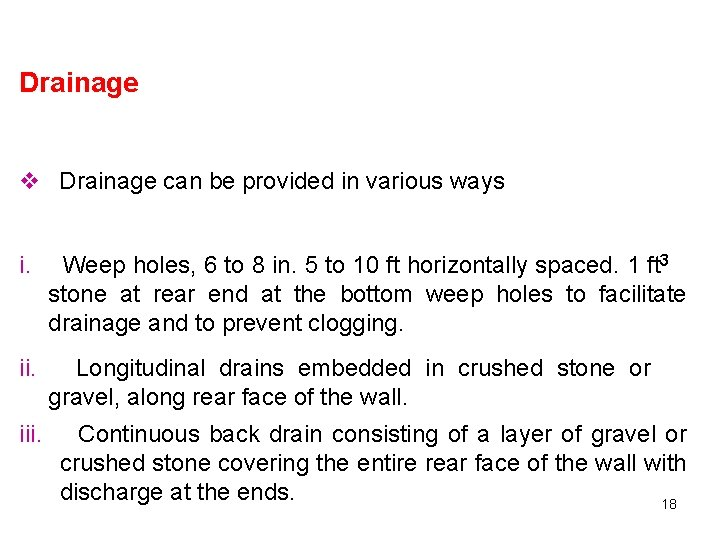Drainage v Drainage can be provided in various ways i. Weep holes, 6 to 8 in. 5 to 10 ft horizontally spaced. 1 ft 3 stone at rear end at the bottom weep holes to facilitate drainage and to prevent clogging. ii. Longitudinal drains embedded in crushed stone or gravel, along rear face of the wall. iii. Continuous back drain consisting of a layer of gravel or crushed stone covering the entire rear face of the wall with discharge at the ends. 18Estimating size of cantilever retaining wall Height of Wall Ø The base of footing should be below frost penetration about 3’ or 4’. Stem Thickness. Ø Stem is thickest at its base. They have thickness in the range of 8 to 12% of overall height of the retaining wall. The minimum thickness at the top is 10” but preferably 12”. Base Thickness Ø Preferably, total thickness of base fall between 7 and 10% of the overall wall height. Minimum thickness is at least 10” to 1912” used.Estimating size of cantilever retaining wall Ø Base Length For preliminary estimates, the base length can be taken about 40 to 60% of the overall wall height. Ø Another method refer to fig. W is assumed to be equal to weight of the material within area abcd. Ø Take moments about toe and solve for x. 20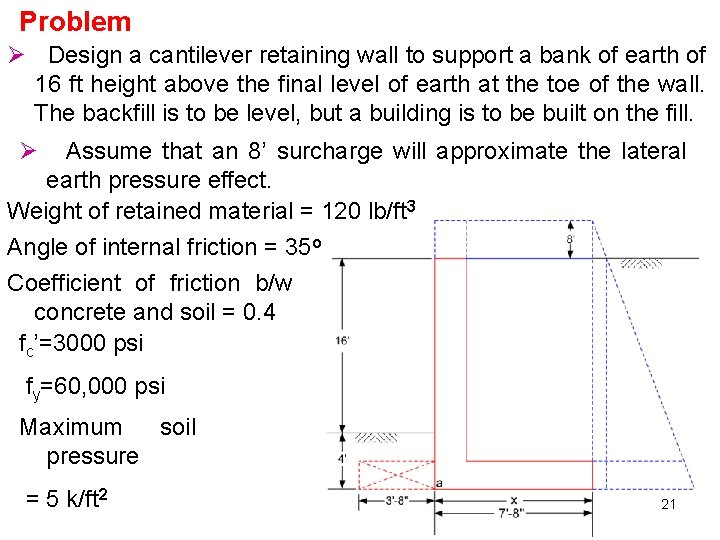Problem Ø Design a cantilever retaining wall to support a bank of earth of 16 ft height above the final level of earth at the toe of the wall. The backfill is to be level, but a building is to be built on the fill. Ø Assume that an 8’ surcharge will approximate the lateral earth pressure effect. Weight of retained material = 120 lb/ft 3 Angle of internal friction = 35 o Coefficient of friction b/w concrete and soil = 0. 4 fc’=3000 psi fy=60, 000 psi Maximum soil pressure = 5 k/ft 2 21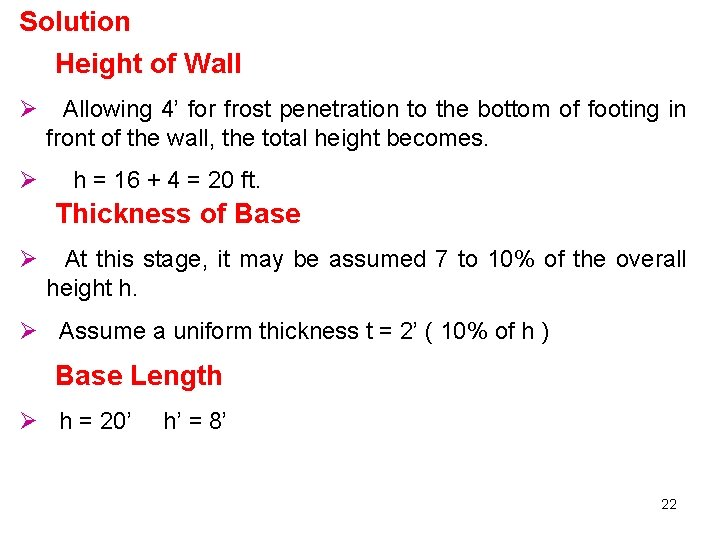Solution Height of Wall Ø Allowing 4’ for frost penetration to the bottom of footing in front of the wall, the total height becomes. Ø h = 16 + 4 = 20 ft. Thickness of Base Ø At this stage, it may be assumed 7 to 10% of the overall height h. Ø Assume a uniform thickness t = 2’ ( 10% of h ) Base Length Ø h = 20’ h’ = 8’ 22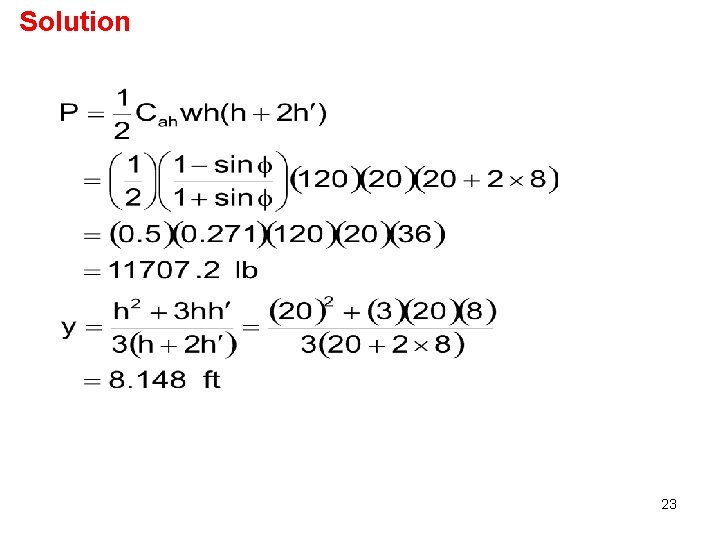Solution 23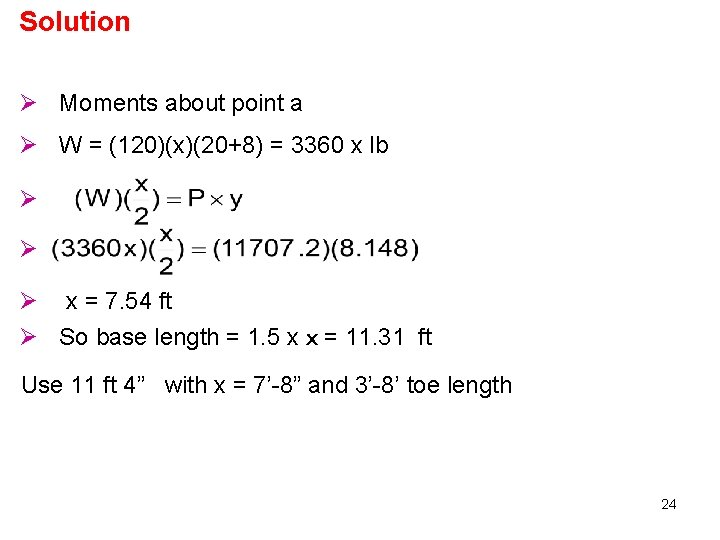Solution Ø Moments about point a Ø W = (120)(x)(20+8) = 3360 x lb Ø Ø Ø x = 7. 54 ft Ø So base length = 1. 5 x x = 11. 31 ft Use 11 ft 4” with x = 7’-8” and 3’-8’ toe length 24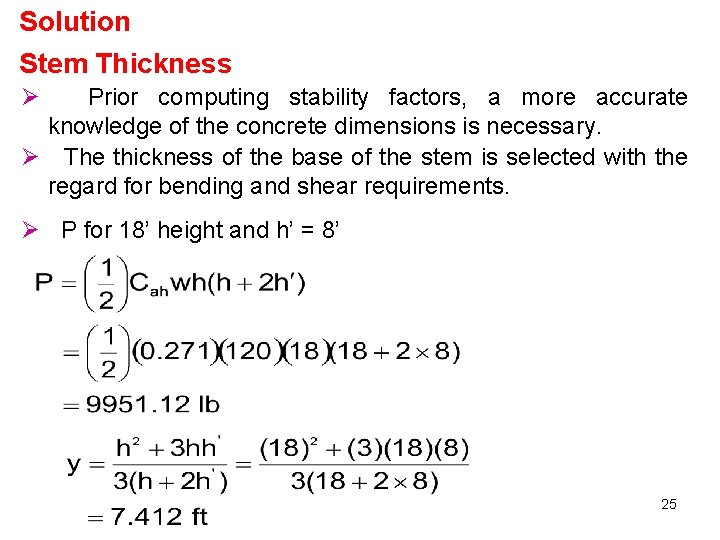Solution Stem Thickness Ø Prior computing stability factors, a more accurate knowledge of the concrete dimensions is necessary. Ø The thickness of the base of the stem is selected with the regard for bending and shear requirements. Ø P for 18’ height and h’ = 8’ 25Solution Ø Mu = (1. 6) Py = (1. 6) (9951. 12) (7. 412) = 118004 lb. ft For economy and ease of bar placement, 26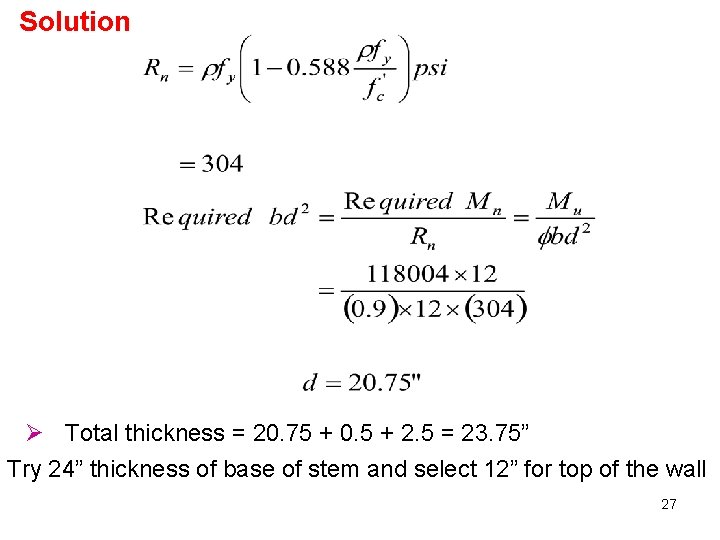Solution Ø Total thickness = 20. 75 + 0. 5 + 2. 5 = 23. 75” Try 24” thickness of base of stem and select 12” for top of the wall 27Solution Shear at d d used now = 24 -0. 5 -2. 5=21”=1. 75’ Ø At 18’ – 1. 75’ = 16. 25’ from top 28Solution F. O. S Against Overturning Component W 1 W 2 Force Arm Moment (5. 667)(18)(120)=12239. 7 3. 67+2+5. 667/2 104037. 9 3. 67+1+1/3 6750. 6 (1)(18)(150)=1350. 1 W 3 (18)(1)(150)=2700 3. 67+0. 5=4. 17 11250 W 4 (11. 33)(2)(150)=3399. 00 11. 33/2 19267 W 5 (18)(1)(120)=1080 3. 67+1+2/3 4187. 7 3. 67+1+6. 67/2 51200 W 6 Total (6. 67)(8)(120)=6400 27170. 1 198266. 6 29Solution Ø P = 11707. 2 lb y= 8. 148 ft Ø Overturning Moment = 11707. 2 x 8. 148 = 95390. 27 lb. ft F. O. S. against overturning O. K Location of Resultant & Footing Soil Pressure Ø Distance of the resultant from the front edge is Ø Middle third=L/3= 3. 77 ft, a>L/3, So resultant is within the middle third. 30Solution q 1= 4783. 7 lb/ft 2 < 5 k/ft 2 So O. K against bearing pressure. 31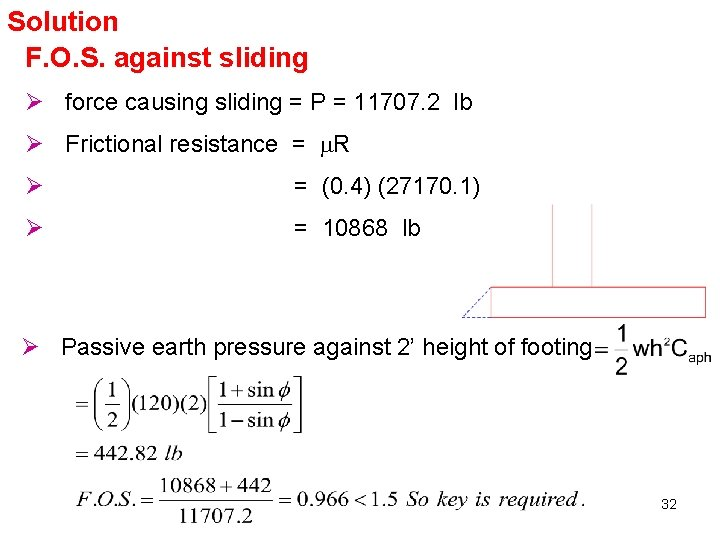Solution F. O. S. against sliding Ø force causing sliding = P = 11707. 2 lb Ø Frictional resistance = R Ø = (0. 4) (27170. 1) Ø = 10868 lb Ø Passive earth pressure against 2’ height of footing 32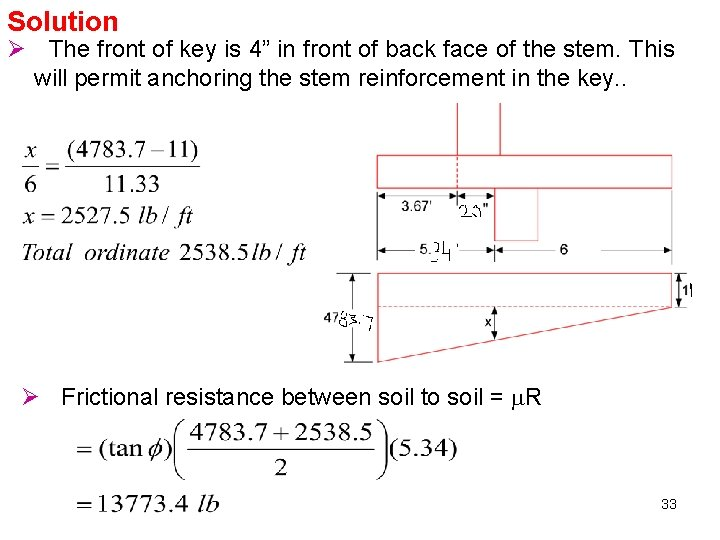Solution Ø The front of key is 4” in front of back face of the stem. This will permit anchoring the stem reinforcement in the key. . Ø Frictional resistance between soil to soil = R 33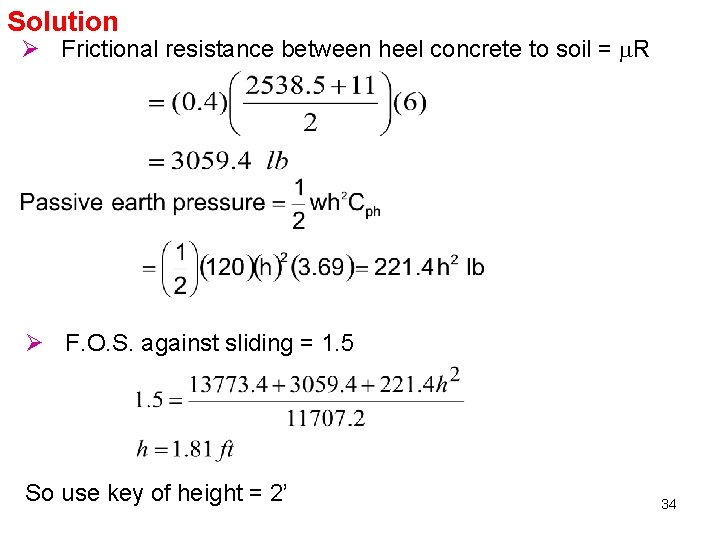Solution Ø Frictional resistance between heel concrete to soil = R Ø F. O. S. against sliding = 1. 5 So use key of height = 2’ 34Solution Design of Heel Cantilever Wu = (1. 6) (120) (8) + (1. 2) [18 x 120 + 2 x 150 ] = 4488 lb/ft Vu = Factored shear a joint of stem and heel When the support reaction introduces compression into the end region, then critical shear is at a distance d from face of support. However, the support is not producing compression, therefore, critical shear is at joint of stem and heel. 35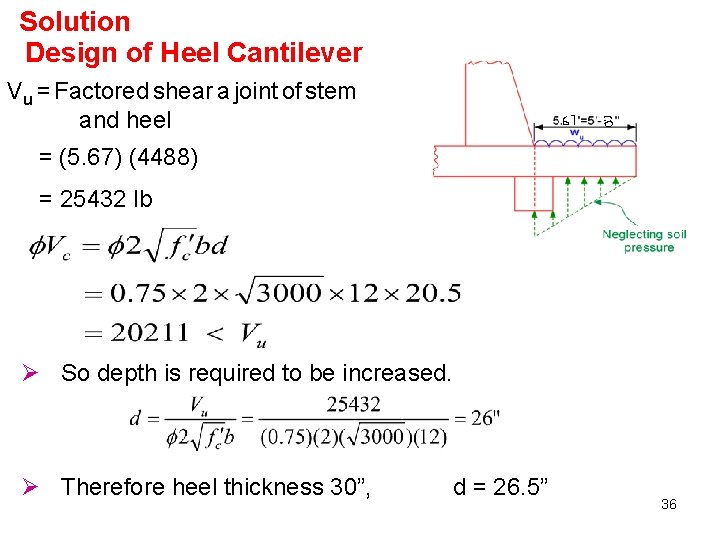Solution Design of Heel Cantilever Vu = Factored shear a joint of stem and heel = (5. 67) (4488) = 25432 lb Ø So depth is required to be increased. Ø Therefore heel thickness 30”, d = 26. 5” 36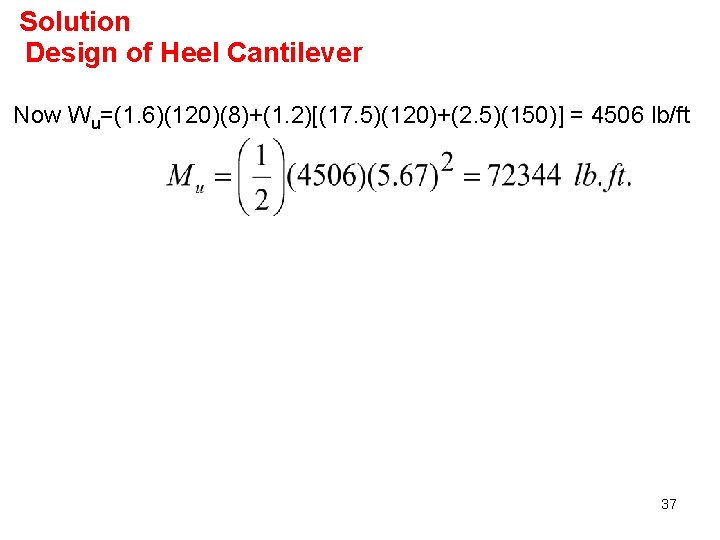Solution Design of Heel Cantilever Now Wu=(1. 6)(120)(8)+(1. 2)[(17. 5)(120)+(2. 5)(150)] = 4506 lb/ft 37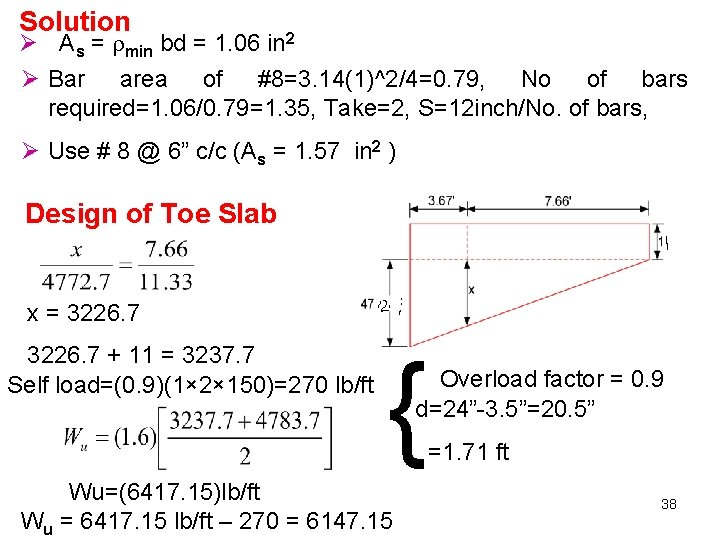Solution Ø As = min bd = 1. 06 in 2 Ø Bar area of #8=3. 14(1)^2/4=0. 79, No of bars required=1. 06/0. 79=1. 35, Take=2, S=12 inch/No. of bars, Ø Use # 8 @ 6” c/c (As = 1. 57 in 2 ) Design of Toe Slab x = 3226. 7 + 11 = 3237. 7 Self load=(0. 9)(1× 2× 150)=270 lb/ft { Wu=(6417. 15)lb/ft Wu = 6417. 15 lb/ft – 270 = 6147. 15 Overload factor = 0. 9 d=24”-3. 5”=20. 5” =1. 71 ft 38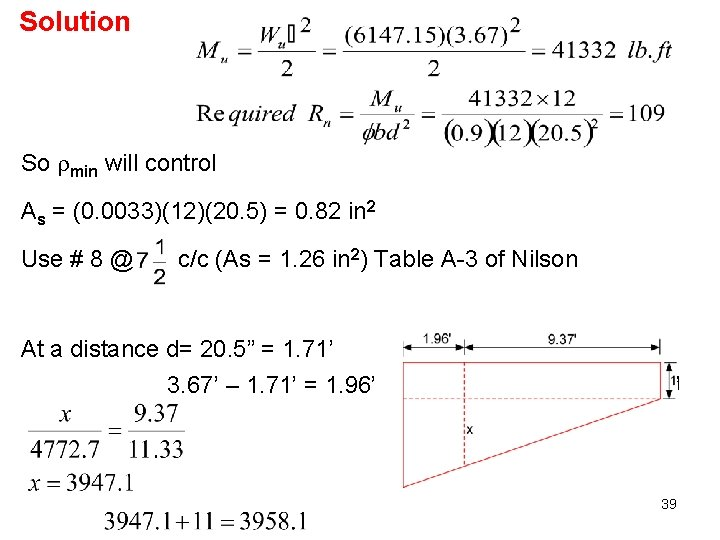Solution So min will control As = (0. 0033)(12)(20. 5) = 0. 82 in 2 Use # 8 @ c/c (As = 1. 26 in 2) Table A-3 of Nilson At a distance d= 20. 5” = 1. 71’ 3. 67’ – 1. 71’ = 1. 96’ 39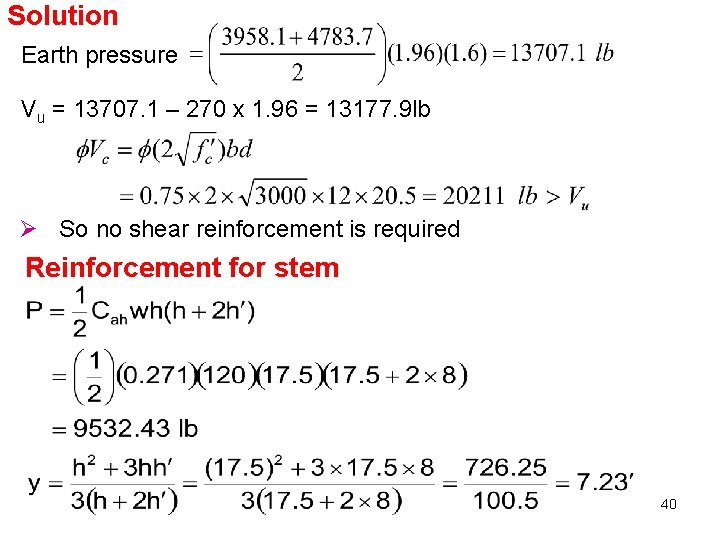Solution Earth pressure Vu = 13707. 1 – 270 x 1. 96 = 13177. 9 lb Ø So no shear reinforcement is required Reinforcement for stem 40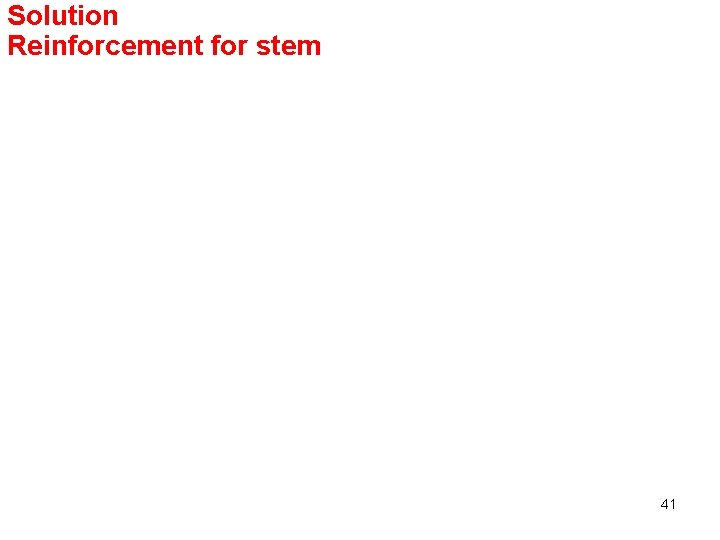Solution Reinforcement for stem 41Solution Temperature & shrinkage reinforcement Ø Total amount of horizontal bars (h is average thickness) Ø Since front face is more exposed for temperature changes therefore two third of this amount is placed in front face and one third in rear face. Accordingly Ø # 4 @ 8 in. c/c As=0. 29 in 2. Use # 3 @ 9 in. c/c As=0. 15 in 2. Ø For vertical reinforcement on the front face, use any nominal amount. Use # 3 @ 18 in. c/c Since base is not subjected to extreme temperature changes, 42 therefore # [email protected] 12” c/c just for spacers will be sufficient.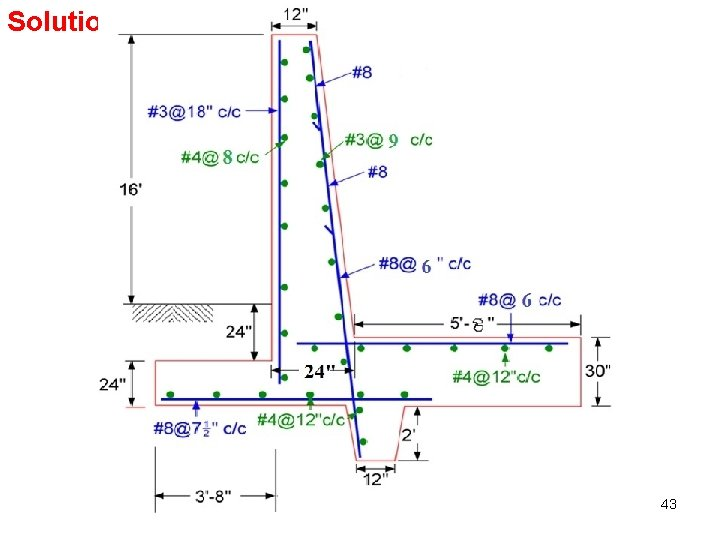Solution 43